# Graphical Solutions of Linear Programming Models

[Page 34 ( continued )]

Following the formulation of a mathematical model, the next stage in the application of linear programming to a decision-making problem is to find the solution of the model. A common solution approach is to solve algebraically the set of mathematical relationships that form the model either manually or using a computer program, thus determining the values for the decision variables . However, because the relationships are linear , some models and solutions can be illustrated graphically .

Graphical solutions are limited to linear programming problems with only two decision variables .

The graphical method is realistically limited to models with only two decision variables, which can be represented on a graph of two dimensions. Models with three decision variables can be graphed in three dimensions, but the process is quite cumbersome, and models of four or more decision variables cannot be graphed at all.

Although the graphical method is limited as a solution approach, it is very useful at this point in our presentation of linear programming in that it gives a picture of how a solution is derived. Graphs can provide a clearer understanding of how the computer and mathematical solution approaches presented in subsequent chapters work and, thus, a better understanding of the solutions.

The graphical method provides a picture of how a solution is obtained for a linear programming problem.

#### Graphical Solution of a Maximization Model

The product mix model will be used to demonstrate the graphical interpretation of a linear programming problem. Recall that the problem describes Beaver Creek Pottery Company's attempt to decide how many bowls and mugs to produce daily, given limited amounts of labor and clay. The complete linear programming model was formulated as

maximize Z = \$40 x 1 + 50 x 2

subject to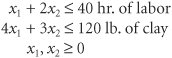where

x 1 = number of bowls produced

x 2 = number of mugs produced

Figure 2.2 is a set of coordinates for the decision variables x 1 and x 2 , on which the graph of our model will be drawn. Note that only the positive quadrant is drawn (i.e., the quadrant where x 1 and x 2 will always be positive) because of the nonnegativity constraints, x 1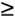0 and x 20.

[Page 35]

## Management Science Application: Operational Cost Control at Kellogg

Kellogg is the world's largest cereal producer and a leading producer of convenience foods , with worldwide sales in 1999 of almost \$7 billion. The company started with a single product, Kellogg's Corn Flakes, in 1906 and over the years developed a product line of other cereals, including Rice Krispies and Corn Pops, and convenience foods such as Pop-Tarts and Nutri-Grain cereal bars. Kellogg operates 5 plants in the United States and Canada and 7 distribution centers, and it contracts with 15 co-packers to produce or pack some of the Kellogg products. Kellogg must coordinate the production, packaging, inventory, and distribution of roughly 80 cereal products alone at these various facilities.

For more than a decade Kellogg has been using a large-scale linear programming model called the Kellogg Planning System (KPS) to plan its weekly production, inventory, and distribution decisions. The model decision variables include the amount of each product produced in a production process at each plant, the units of product packaged, the amount of inventory held, and the shipments of products to other plants and distribution centers. Model constraints include production processing time, packaging capacity, balancing constraints that make sure that all products produced are also packaged during the week, inventory balancing constraints, and inventory safety stock requirements. The model objective is cost minimization. Kellogg has also developed a tactical version of this basic operational linear programming model for long-range planning for 12 to 24 months into the future. The KPS model is credited with saving Kellogg \$4.5 million in reduced production, inventory, and distribution costs in 1995, and it is estimated that KPS has saved Kellogg many more millions of dollars since the mid-1990s. The tactical version of KPS recently helped the company consolidate production capacity with estimated projected savings of almost \$40 million.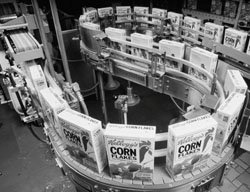Source: G. Brown, J. Keegan, B. Vigus, and K. Wood, "The Kellogg Company Optimizes Production, Inventory, and Distribution," Interfaces 31, no. 6 (NovemberDecember 2001): 115.

##### Figure 2.2. Coordinates for graphical analysis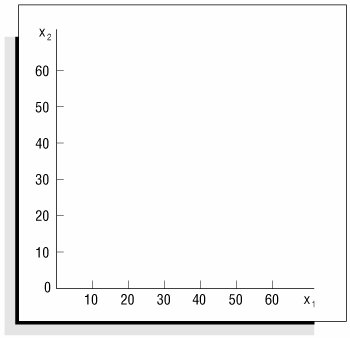[Page 36]

The first step in drawing the graph of the model is to plot the constraints on the graph. This is done by treating both constraints as equations (or straight lines) and plotting each line on the graph. Let's consider the labor constraint line first:

x 1 + 2 x 2 = 40

Constraint lines are plotted as equations .

A simple procedure for plotting this line is to determine two points that are on the line and then draw a straight line through the points. One point can be found by letting x 1 = 0 and solving for x 2 :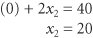Thus, one point is at the coordinates x 1 = 0 and x 2 = 20. A second point can be found by letting x 2 = 0 and solving for x 1 :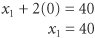Now we have a second point, x 1 = 40, x 2 = 0. The line on the graph representing this equation is drawn by connecting these two points, as shown in Figure 2.3. However, this is only the graph of the constraint line and does not reflect the entire constraint, which also includes the values that are less than or equal to (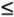) this line. The area representing the entire constraint is shown in Figure 2.4.

##### Figure 2.3. Graph of the labor constraint line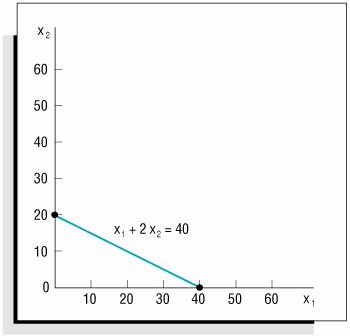To test the correctness of the constraint area, we check any two pointsone inside the constraint area and one outside. For example, check point A in Figure 2.4, which is at the intersection of x 1 = 10 and x 2 = 10. Substituting these values into the following labor constraint,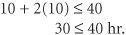[Page 37]

shows that point A is indeed within the constraint area, as these values for x 1 and x 2 yield a quantity that does not exceed the limit of 40 hours. Next, we check point B at x 1 = 40 and x 2 = 30: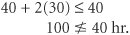##### Figure 2.4. The labor constraint area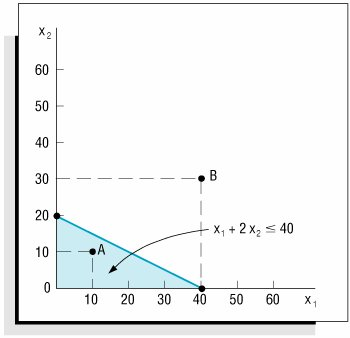Point B is obviously outside the constraint area because the values for x 1 and x 2 yield a quantity (100) that exceeds the limit of 40 hours.

We draw the line for the clay constraint the same way as the one for the labor constraintby finding two points on the constraint line and connecting them with a straight line. First, let x 1 = 0 and solve for x 2 :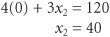Performing this operation results in a point, x 1 = 0, x 2 = 40. Next, we let x 2 = 0 and then solve for x 1 :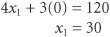This operation yields a second point, x 1 = 30, x 2 = 0. Plotting these points on the graph and connecting them with a line gives the constraint line and area for clay, as shown in Figure 2.5.

##### (This item is displayed on page 38 in the print version)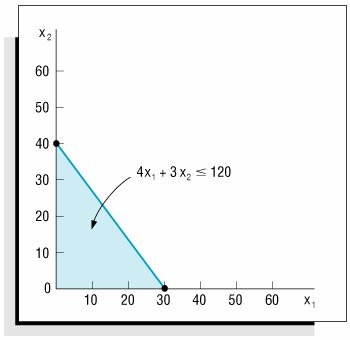Combining the two individual graphs for both labor and clay (Figures 2.4 and 2.5) produces a graph of the model constraints, as shown in Figure 2.6. The shaded area in Figure 2.6 is the area that is common to both model constraints. Therefore, this is the only area on the graph that contains points (i.e., values for x 1 and x 2 ) that will satisfy both constraints simultaneously . For example, consider the points R , S , and T in Figure 2.7. Point R satisfies both constraints; thus, we say it is a feasible solution point. Point S satisfies the clay constraint (4 x 1 + 3 x 2120) but exceeds the labor constraint; thus, it is infeasible. Point T satisfies neither constraint; thus, it is also infeasible.

[Page 38]
##### Figure 2.6. Graph of both model constraints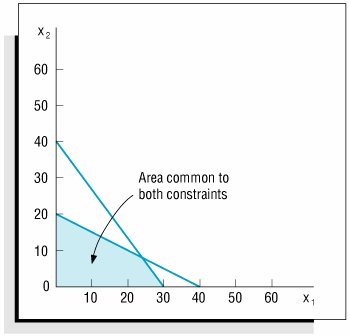The shaded area in Figure 2.7 is referred to as the feasible solution area because all the points in this area satisfy both constraints. Some point within this feasible solution area will result in maximum profit for Beaver Creek Pottery Company. The next step in the graphical solution approach is to locate this point.

The feasible solution area is an area on the graph that is bounded by the constraint equations .

#### The Optimal Solution Point

The second step in the graphical solution method is to locate the point in the feasible solution area that will result in the greatest total profit. To begin the solution analysis, we first plot the objective function line for an arbitrarily selected level of profit. For example, if we say profit, Z , is \$800, the objective function is

\$800 = 40 x 1 + 50 x 2

Plotting this line just as we plotted the constraint lines results in the graph shown in Figure 2.8. Every point on this line is in the feasible solution area and will result in a profit of \$800 (i.e., every combination of x 1 and x 2 on this line will give a Z value of \$800). However, let us see whether an even greater profit will still provide a feasible solution. For example, consider profits of \$1,200 and \$1,600, as shown in Figure 2.9.

[Page 39]
##### Figure 2.7. The feasible solution area constraints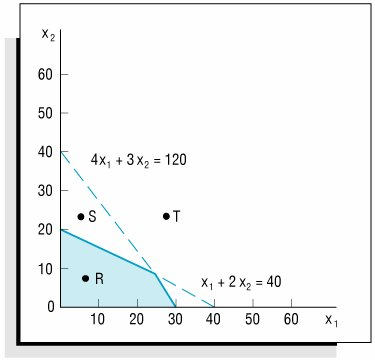##### Figure 2.8. Objective function line for Z = \$800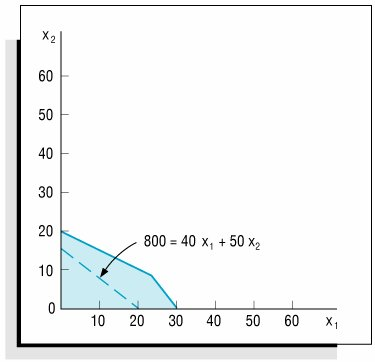A portion of the objective function line for a profit of \$1,200 is outside the feasible solution area, but part of the line remains within the feasible area. Therefore, this profit line indicates that there are feasible solution points that give a profit greater than \$800. Now let us increase profit again, to \$1,600. This profit line, also shown in Figure 2.9, is completely outside the feasible solution area. The fact that no points on this line are feasible indicates that a profit of \$1,600 is not possible.

Because a profit of \$1,600 is too great for the constraint limitations, as shown in Figure 2.9, the question of the maximum profit value remains. We can see from Figure 2.9 that profit increases as the objective function line moves away from the origin (i.e., the point x 1 = 0, x 2 = 0). Given this characteristic, the maximum profit will be attained at the point where the objective function line is farthest from the origin and is still touching a point in the feasible solution area. This point is shown as point B in Figure 2.10.

[Page 40]
##### Figure 2.9. Alternative objective function lines for profits, Z , of \$800, \$1,200, and \$1,600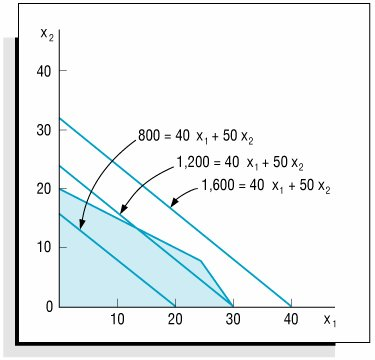##### Figure 2.10. Identification of optimal solution point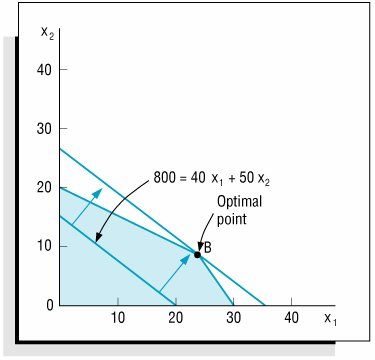To find point B , we place a straightedge parallel to the objective function line \$800 = 40 x 1 + 50 x 2 in Figure 2.10 and move it outward from the origin as far as we can without losing contact with the feasible solution area. Point B is referred to as the optimal (i.e., best) solution.

The optimal solution is the best feasible solution .

#### The Solution Values

The third step in the graphical solution approach is to solve for the values of x 1 and x 2 once the optimal solution point has been found. It is possible to determine the x 1 and x 2 coordinates of point B in Figure 2.10 directly from the graph, as shown in Figure 2.11. The graphical coordinates corresponding to point B in Figure 2.11 are x 1 = 24 and x 2 = 8. This is the optimal solution for the decision variables in the problem. However, unless an absolutely accurate graph is drawn, it is frequently difficult to determine the correct solution directly from the graph. A more exact approach is to determine the solution values mathematically once the optimal point on the graph has been determined. The mathematical approach for determining the solution is described in the following pages. First, however, we will consider a few characteristics of the solution.

[Page 41]
##### Figure 2.11. Optimal solution coordinates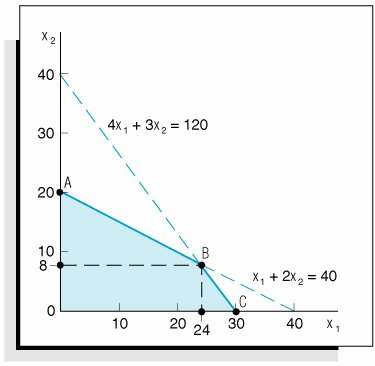In Figure 2.10, as the objective function was increased, the last point it touched in the feasible solution area was on the boundary of the feasible solution area. The solution point is always on this boundary because the boundary contains the points farthest from the origin (i.e., the points corresponding to the greatest profit). This characteristic of linear programming problems reduces the number of possible solution points considerably, from all points in the solution area to just those points on the boundary. However, the number of possible solution points is reduced even more by another characteristic of linear programming problems.

The optimal solution point is the last point the objective function touches as it leaves the feasible solution area .

The solution point will be on the boundary of the feasible solution area and at one of the corners of the boundary where two constraint lines intersect. (The graphical axes, you will recall, are also constraints because x 10 and x 20.) These corners (points A , B , and C in Figure 2.11) are protrusions, or extremes , in the feasible solution area; they are called extreme points . It has been proven mathematically that the optimal solution in a linear programming model will always occur at an extreme point. Therefore, in our sample problem the possible solution points are limited to the three extreme points, A , B , and C . The optimal extreme point is the extreme point the objective function touches last as it leaves the feasible solution area, as shown in Figure 2.10.

Extreme points are corner points on the boundary of the feasible solution area .

From the graph shown in Figure 2.10, we know that the optimal solution point is B . Because point B is formed by the intersection of two constraint lines, as shown in Figure 2.11, these two lines are equal at point B . Thus, the values of x 1 and x 2 at that intersection can be found by solving the two equations simultaneously .

Constraint equations are solved simultaneously at the optimal extreme point to determine the variable solution values .

First, we convert both equations to functions of x 1 :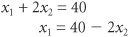and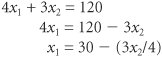[Page 42]

Now we let x 1 in the first equation equal x 1 in the second equation,

40 2 x 2 = 30 (3 x 2 /4)

and solve for x 2 :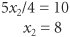Substituting x 2 = 8 into either one of the original equations gives a value for x 1 :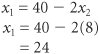Thus, the optimal solution at point B in Figure 2.11 is x 1 = 24 and x 2 = 8. Substituting these values into the objective function gives the maximum profit,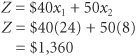In terms of the original problem, the solution indicates that if the pottery company produces 24 bowls and 8 mugs, it will receive \$1,360, the maximum daily profit possible (given the resource constraints).

Given that the optimal solution will be at one of the extreme corner points, A , B , or C , we can also find the solution by testing each of the three points to see which results in the greatest profit, rather than by graphing the objective function and seeing which point it last touches as it moves out of the feasible solution area. Figure 2.12 shows the solution values for all three points, A , B , and C , and the amount of profit, Z , at each point.

##### Figure 2.12. Solutions at all corner points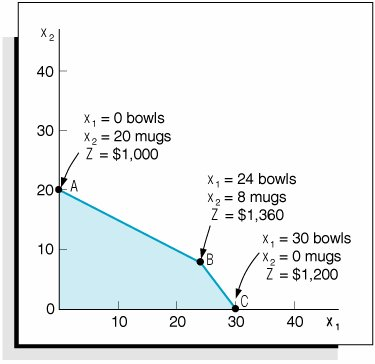As indicated in the discussion of Figure 2.10, point B is the optimal solution point because it is the last point the objective function touches before it leaves the solution area. In other words, the objective function determines which extreme point is optimal. This is because the objective function designates the profit that will accrue from each combination of x 1 and x 2 values at the extreme points. If the objective function had had different coefficients (i.e., different x 1 and x 2 profit values), one of the extreme points other than B might have been optimal.

[Page 43]

Let's assume for a moment that the profit for a bowl is \$70 instead of \$40, and the profit for a mug is \$20 instead of \$50. These values result in a new objective function, Z = \$70 x 1 + 20 x 2 . If the model constraints for labor or clay are not changed, the feasible solution area remains the same, as shown in Figure 2.13. However, the location of the objective function in Figure 2.13 is different from that of the original objective function in Figure 2.10. The reason for this change is that the new profit coefficients give the linear objective function a new slope .

##### Figure 2.13. The optimal solution with Z = 70 x 1 + 20 x 2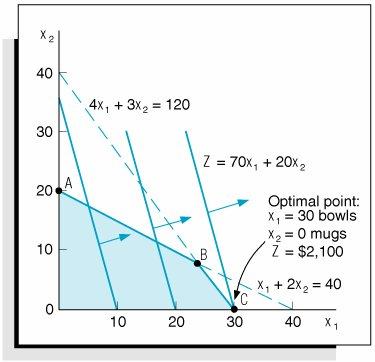The slope can be determined by transforming the objective function into the general equation for a straight line, y = a + bx , where y is the dependent variable, a is the y intercept, b is the slope, and x is the independent variable. For our sample objective function, x 2 is the dependent variable corresponding to y (i.e., it is on the vertical axis), and x 1 is the independent variable. Thus, the objective function can be transformed into the general equation of a line as follows :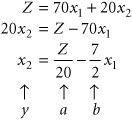The slope is computed as the "rise" over the "run."

This transformation identifies the slope of the new objective function as 7/2 (the minus sign indicates that the line slopes downward). In contrast, the slope of the original objective function was 4/5.

If we move this new objective function out through the feasible solution area, the last extreme point it touches is point C . Simultaneously solving the constraint lines at point C results in the following solution: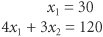and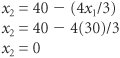Thus, the optimal solution at point C in Figure 2.13 is x 1 = 30 bowls, x 2 = 0 mugs, and Z = \$2,100 profit. Altering the objective function coefficients results in a new solution.

[Page 44]

This brief example of the effects of altering the objective function highlights two useful points. First, the optimal extreme point is determined by the objective function, and an extreme point on one axis of the graph is as likely to be the optimal solution as is an extreme point on a different axis. Second, the solution is sensitive to the values of the coefficients in the objective function. If the objective function coefficients are changed, as in our example, the solution may change. Likewise, if the constraint coefficients are changed, the solution space and solution points may change also. This information can be of consequence to the decision maker trying to determine how much of a product to produce. Sensitivity analysis the use of linear programming to evaluate the effects of changes in model parametersis discussed in Chapter 3.

Sensitivity analysis is used to analyze changes in model parameters .

It should be noted that some problems do not have a single extreme point solution. For example, when the objective function line parallels one of the constraint lines, an entire line segment is bounded by two adjacent corner points that are optimal; there is no single extreme point on the objective function line. In this situation there are multiple optimal solutions . This and other irregular types of solution outcomes in linear programming are discussed at the end of this chapter.

Multiple optimal solutions can occur when the objective function is parallel to a constraint line .

#### Slack Variables

Once the optimal solution was found at point B in Figure 2.12, simultaneous equations were solved to determine the values of x 1 and x 2 . Recall that the solution occurs at an extreme point where constraint equation lines intersect with each other or with the axis. Thus, the model constraints are considered as equations (=) rather thanorinequalities .

There is a standard procedure for transforminginequality constraints into equations. This transformation is achieved by adding a new variable, called a slack variable , to each constraint. For the pottery company example, the model constraints are

x 1 + 2 x 240 hr. of labor

4 x 1 + 3 x 2120 lb. of clay

A slack variable is added to aconstraint to convert it to an equation (=) .

The addition of a unique slack variable, s 1 , to the labor constraint and s 2 to the constraint for clay results in the following equations:

x 1 + 2 x 2 + s 1 = 40 hr. of labor

4 x 1 + 3 x 2 + s 2 = 120 lb. of clay

A slack variable represents unused resources .

The slack variables in these equations, s 1 and s 2 , will take on any value necessary to make the left-hand side of the equation equal to the right-hand side. For example, consider a hypothetical solution of x 1 = 5 and x 2 = 10. Substituting these values into the foregoing equations yields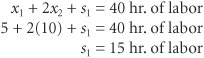and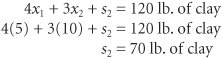In this example, x 1 = 5 bowls and x 2 = 10 mugs represent a solution that does not make use of the total available amount of labor and clay. In the labor constraint, 5 bowls and 10 mugs require only 25 hours of labor. This leaves 15 hours that are not used. Thus, s 1 represents the amount of unused labor, or slack.

[Page 45]

In the clay constraint, 5 bowls and 10 mugs require only 50 pounds of clay. This leaves 70 pounds of clay unused. Thus, s 2 represents the amount of unused clay. In general, slack variables represent the amount of unused resources .

The ultimate instance of unused resources occurs at the origin, where x 1 = 0 and x 2 = 0. Substituting these values into the equations yields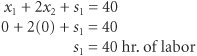and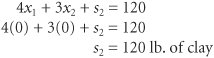Because no production takes place at the origin, all the resources are unused; thus, the slack variables equal the total available amounts of each resource: s 1 = 40 hours of labor and s 2 = 120 pounds of clay.

What is the effect of these new slack variables on the objective function? The objective function for our example represents the profit gained from the production of bowls and mugs,

Z = \$40 x 1 + \$50 x 2

The coefficient \$40 is the contribution to profit of each bowl; \$50 is the contribution to profit of each mug. What, then, do the slack variables s 1 and s 2 contribute? They contribute nothing to profit because they represent unused resources. Profit is made only after the resources are put to use in making bowls and mugs. Using slack variables, we can write the objective function as

A slack variable contributes nothing to the objective function value.

maximize Z = \$40 x 1 + \$50 x 2 + 0 s 1 + 0 s 2

As in the case of decision variables ( x 1 and x 2 ), slack variables can have only nonnegative values because negative resources are not possible. Therefore, for this model formulation, x 1 , x 2 , s 1 , and s 20.

The complete linear programming model can be written in what is referred to as standard form with slack variables as follows: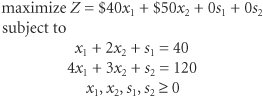The solution values, including the slack at each solution point, are summarized as follows:

Solution Summary with Slack

Point

Solution Values

Z

Slack

A

x 1 = 0 bowls, x 2 = 20 mugs

\$1,000

s 1 = 0 hr.; s 2 = 60 lb.

B

x 1 = 24 bowls, x 2 = 8 mugs

\$1,360

s 1 = 0 hr.; s 2 = 0 lb.

C

x 1 = 30 bowls, x 2 = 0 mugs

\$1,200

s 1 = 10 hr.; s 2 = 0 lb.

Figure 2.14 shows the graphical solution of this example, with slack variables included at each solution point.

[Page 46]
##### Figure 2.14. Solutions at points A , B , and C with slack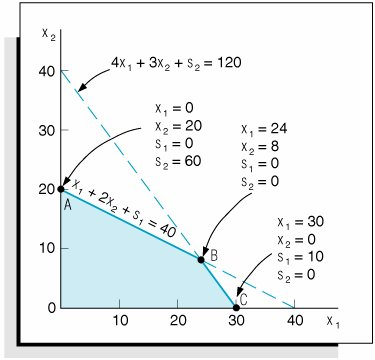## Management Science Application: Estimating Food Nutrient Values at Minnesota's Nutrition Coordinating Center

The Nutrition Coordinating Center (NCC) at the University of Minnesota maintains a food composition database used by institutions around the world. This database is used to calculate dietary material intake; to plan menus ; to explore the relationships between diet and disease; to meet regulatory requirements; and to monitor the effects of education, intervention, and regulation. These calculations require an enormous amount of nutrient value data for different food products.

Every year, more than 12,000 new brand- name food products are introduced into the marketplace . However, it's not feasible to perform a chemical analysis on all foods for multiple nutrients, and so the NCC estimates thousands of nutrient values each year. The NCC has used a time-consuming trial-and-error approach to estimating these nutrient values. These estimates are a composite of the foods already in the database. The NCC developed a linear programming model that determines ingredient amounts in new food products. These estimated ingredient amounts are subsequently used to calculate nutrient amounts for a food product. The nutritionist normally uses the linear programming model to derive an initial estimate of ingredient amounts and then fine-tunes these amounts. The model has reduced the average time it takes to estimate a product formula from 8.3 minutes (using the trial-and-error approach) to 2.1 minutes of labor time and effort.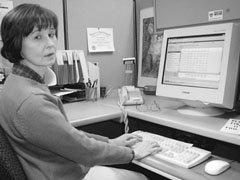Source: B. J. Westrich, M. A. Altmann, and S. J. Potthoff, "Minnesota's Nutrition Coordinating Center Uses Mathematical Optimization to Estimate Food Nutrient Values," Interfaces 28, no. 5 (SeptemberOctober 1998): 8699.

#### Summary of the Graphical Solution Steps

The steps for solving a graphical linear programming model are summarized here:

 1. Plot the model constraints as equations on the graph; then, considering the inequalities of the constraints, indicate the feasible solution area. [Page 47] 2. Plot the objective function; then move this line out from the origin to locate the optimal solution point. 3. Solve simultaneous equations at the solution point to find the optimal solution values.

Or

 2 Solve simultaneous equations at each corner point to find the solution values at each point. 3 Substitute these values into the objective function to find the set of values that results in the maximum Z value.Introduction to Management Science (10th Edition)
ISBN: 0136064361
EAN: 2147483647
Year: 2006
Pages: 358

Similar book on Amazon

flylib.com © 2008-2017.
If you may any questions please contact us: flylib@qtcs.net